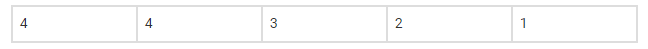# A five digit number divisible by 3 is to be formed using the numbers 0, 1, 2, 3, 4`
Question:

A five digit number divisible by 3 is to be formed using the numbers 0, 1, 2, 3, 4 and 5 without repetitions. The total number of ways this can be done is
A. 216
B. 600
C. 240
D. 3125
[Hint:5 digit numbers can be formed using digits 0, 1, 2, 4, 5 or by using digits 1, 2, 3, 4, 5 since sum of digits in these cases is divisible by 3.]

Solution:

A. 216

Explanation:

5-digit numbers that can be formed using digits 0, 1, 2, 4, 54×4×3×2×1=96

5-digit numbers can be formed using digits 1, 2, 3, 4, 5 = 5!

Total number of ways = 5! +96

=216

Hence, Option (A) 216 is the correct answer.